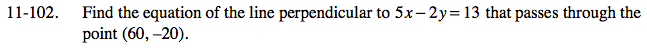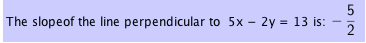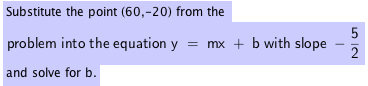Home > AC > Chapter 11 > Lesson 11.3.1 > Problem11-102

11-102.

Find the equation of the line perpendicular to 5x − 2y = 13 that passes through the point (60, −20). Homework Help ✎Solve for y so the equation is in y = mx + b form. m = slope (growth)

Subtract 5x from both sides.

−2y = −5x + 13

Divide both sides by −2.

$y = \frac{ -2 }{ -5} x + \frac{ 13 }{ -5}$

$y = \frac{ 2 }{5 } x - \frac{ 13 }{ 5}$

The line perpendicular to the line in the problem has a slope that is the negative reciprocal of the slope of line.$y = -\frac{ 5 }{ 2} x + b$

$-20 = - \frac{ 5 }{2 }(60) + b$

b = 130

$y = -\frac{5 }{2 } + 130$